Courses

# Short Notes - Motion Class 9 Notes | EduRev

## Class 9: Short Notes - Motion Class 9 Notes | EduRev

The document Short Notes - Motion Class 9 Notes | EduRev is a part of the Class 9 Course Class 9 Science by VP Classes.
All you need of Class 9 at this link: Class 9

• An object is said to be in motion when its position changes with time.
• We describe the location of an object by specifying a reference point. Motion is relative. The total path covered by an object is said to be the distance travelled by it.
• The shortest path/distance measured from the initial to the final position of an object is known as the displacement.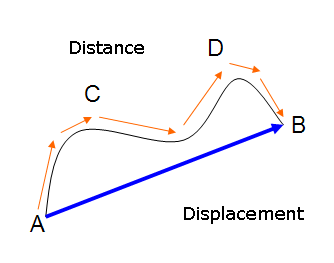• Uniform motion: When an object covers equal distances in equal intervals of time, it is said to be in uniform motion.
• Non-uniform motion: Motions where objects cover unequal distances in equal intervals of time.
• Speed: The distance travelled by an object in unit time is referred to as speed. Its unit is m/s.
• Average speed: For non-uniform motion, the average speed of an object is obtained by dividing the total distance travelled by an object by the total time taken.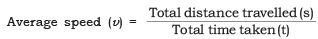• Velocity: Velocity is the speed of an object moving in definite direction. S.I. unit is m/s.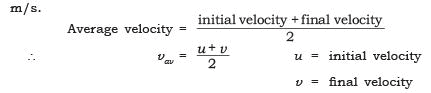• Acceleration: Change in the velocity of an object per unit time.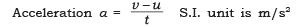• Graphical representation of motion
(i) Distance-time graph: For a distance-time graph time is taken on x-axis and distance is taken on y-axis. [Note: All independent quantities are taken along the x-axis and dependent quantities are taken along y-axis.]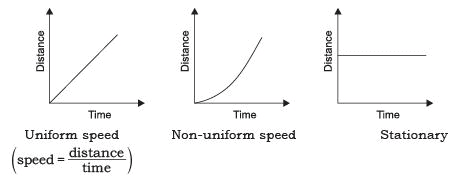• (ii) Velocity-time graph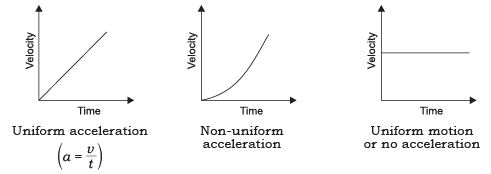• Equations of motion by graphical method
(i) Equation for Velocity-time Relation: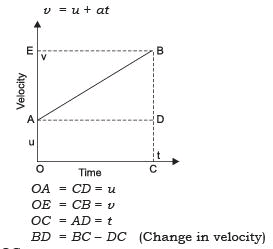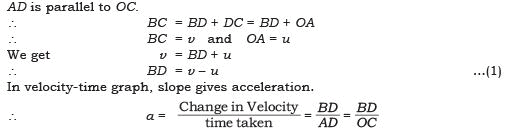(ii) Equation for Position-time Relation: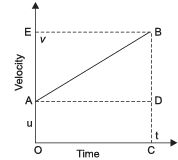Let us assume,
s = distance travelled by the object
t = time t
a = uniform acceleration.
∴ Distance travelled by the object is given by area enclosed with OABC in the graph.
∴ s = area OABC (which is a trapezium)
= (area of rectangle OADC) + (area of ∆ABD)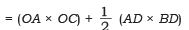Substituting OA = u, OC = AD = t and BD = at (from equation 2)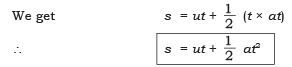(iii) Equation for Position–Velocity Relation: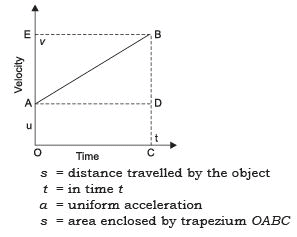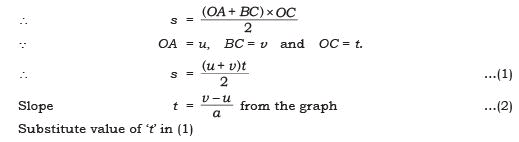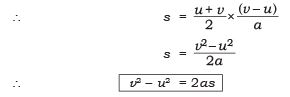• Uniform circular motion: When a body moves in a circular path with uniform speed, its motion is called uniform circular motion.

The document Short Notes - Motion Class 9 Notes | EduRev is a part of the Class 9 Course Class 9 Science by VP Classes.
All you need of Class 9 at this link: Class 9Use Code STAYHOME200 and get INR 200 additional OFF Use Coupon Code
All Tests, Videos & Notes of Class 9: Class 9

### Top Courses for Class 9## Class 9 Science by VP Classes

75 docs|14 tests

### Top Courses for Class 9Track your progress, build streaks, highlight & save important lessons and more!

,

,

,

,

,

,

,

,

,

,

,

,

,

,

,

,

,

,

,

,

,

;